# Statistical Prediction

Monday August 5, 2019

# Logistics

• Final Exam
• Homework 4: Solution

# Part I

Training and testing errors

# Reminder: statistical (regression) models

You have some data $$X_1,\ldots,X_p,Y$$: the variables $$X_1,\ldots,X_p$$ are called predictors, and $$Y$$ is called a response. You’re interested in the relationship that governs them

So you posit that $$Y|X_1,\ldots,X_p \sim P_\theta$$, where $$\theta$$ represents some unknown parameters. This is called regression model for $$Y$$ given $$X_1,\ldots,X_p$$. Goal is to estimate parameters. Why?

• To assess model validity, predictor importance (inference)
• To predict future $$Y$$’s from future $$X_1,\ldots,X_p$$’s (prediction)

# Reminder: linear regression models

The linear model is arguably the most widely used statistical model, has a place in nearly every application domain of statistics

Given response $$Y$$ and predictors $$X_1,\ldots,X_p$$, in a linear regression model, we posit:

$Y = \beta_0 + \beta_1 X_1 + \ldots + \beta_p X_p + \epsilon, \quad \text{where \epsilon \sim N(0,\sigma^2)}$

Goal is to estimate parameters $$\beta_0,\beta_1,\ldots,\beta_p$$. Why?

• To assess whether the linear model is true, which predictors are important (inference)
• To simply predict future $$Y$$’s from future $$X_1,\ldots,X_p$$’s (prediction)

# Good diagnostics, or good predictions?

Nowadays, we try to fit linear models in such a wide variety of difficult problem settings that, in many cases, we have no reason to believe the true data generating model is linear, the errors are close to Gaussian or homoskedastic, etc. Hence, a modern perspective:

The linear model is only a rough approximation, so evaluate prediction accuracy, and let this determine its usefulness

This idea, to focus on prediction, is far more general than linear models. More on this, shortly

# Test error

Suppose we have training data $$X_{i1},\ldots,X_{ip},Y_i$$, $$i=1,\ldots,n$$ used to estimate regression coefficients $$\hat\beta_0,\hat\beta_1,\ldots,\hat\beta_p$$

Given new $$X_1^*,\ldots,X_p^*$$ and asked to predict the associated $$Y^*$$. From the estimated linear model, prediction is: $$\hat{Y^*} = \hat\beta_0 + \hat\beta_1 X_1^* + \ldots + \hat\beta_p X_p^*$$. We define the test error, also called prediction error, by $\mathbb{E}(Y^* - \hat{Y^*})^2$ where the expectation is over every random: training data, $$X_{i1},\ldots,X_{ip},Y_i$$, $$i=1,\ldots,n$$ and test data, $$X_1^*,\ldots,X_p^*,Y^*$$

This was explained for a linear model, but the same definition of test error holds in general

# Estimating test error

Often, we want an accurate estimate of the test error of our method (e.g., linear regression). Why? Two main purposes:

• Predictive assessment: get an absolute understanding of the magnitude of errors we should expect in making future predictions

• Model/method selection: choose among different models/methods, attempting to minimize test error

# Training error

Suppose, as an estimate the test error of our method, we take the observed training error $\frac{1}{n} \sum_{i=1}^n (Y_i - \hat{Y}_i)^2 \quad$

What’s wrong with this? Generally too optimistic as an estimate of the test error—after all, the parameters $$\hat\beta_0,\hat\beta_1,,\ldots,\hat\beta_p$$ were estimated to make $$\hat{Y}_i$$ close to $$Y_i$$, $$i=1,\ldots,n$$, in the first place!

Also, importantly, the more complex/adaptive the method, the more optimistic its training error is as an estimate of test error

# Examples

$X \sim Unif(-3,3) \\ Y \sim 2X + 2*Norm(0, 1)$

set.seed(1)
n <- 30
x <- sort(runif(n, -3, 3))
y <- 2*x + 2*rnorm(n)
x0 <- sort(runif(n, -3, 3))
y0 <- 2*x0 + 2*rnorm(n)

data_vis <- data.frame(x = c(x, x0),
y = c(y, y0),
tt = factor(rep(c("Training data", "Test data"),
each = n),
levels = c("Training data", "Test data")))

ggplot(data_vis) +
geom_point(aes(x = x, y = y)) +
facet_grid(~tt)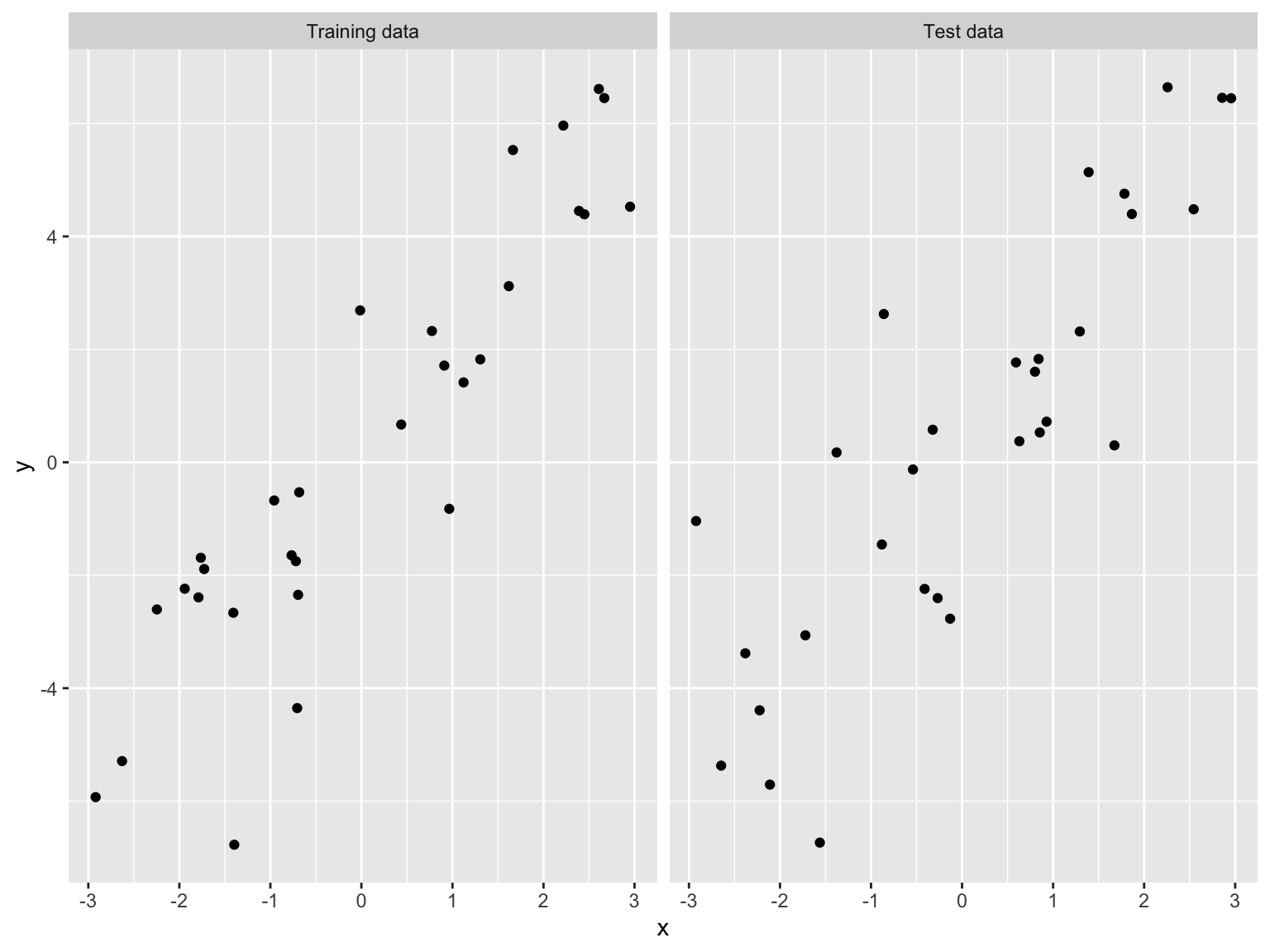# Training and test errors for a simple linear model
lm_1 <- lm(y ~ x)
yhat_1 <- predict(lm_1, data.frame(x = x))
train_err_1 <- mean((y - yhat_1)^2)
y0hat_1 <- predict(lm_1, data.frame(x = x0))
test_err_1 <- mean((y0 - y0hat_1)^2)

data_vis_lm <- data.frame(x = c(x,x0),
yhat = c(yhat_1, y0hat_1),
tt = factor(rep(c("Training data", "Test data"),
each = n)))
ggplot(data_vis) +
geom_point(aes(x = x, y = y)) +
geom_line(data = data_vis_lm, aes(x = x, y = yhat, color = tt)) +
facet_grid(~tt) +
theme(legend.position = "none") +
labs(title = paste0("Training/Test error: ",
round(train_err_1,3) ," / ", round(test_err_1,3)))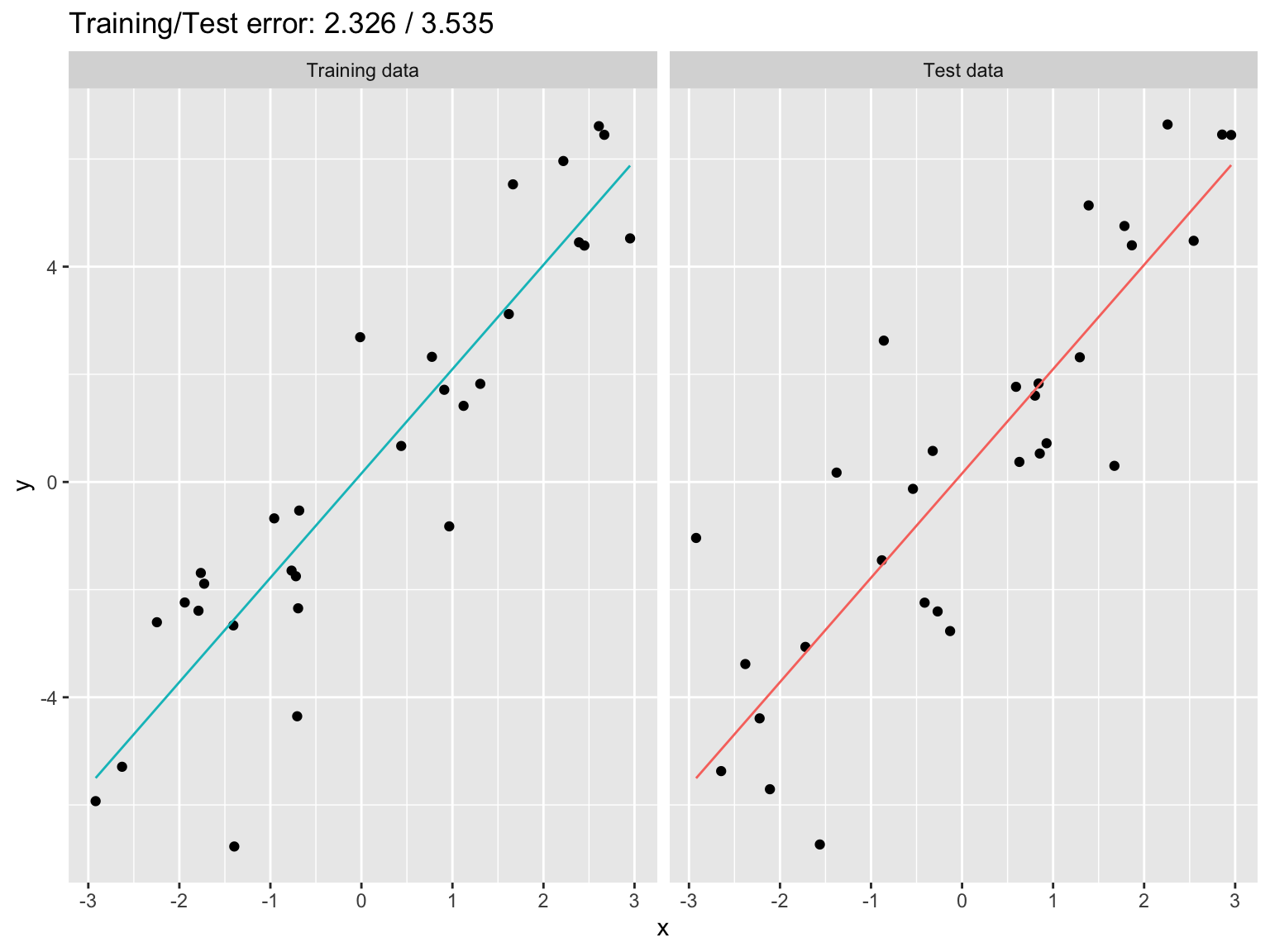# Training and test errors for a 10th order polynomial regression
# (The problem is only exacerbated!)
lm_10 <- lm(y ~ poly(x,10))
yhat_10 <- predict(lm_10, data.frame(x = x))
train_err_10 <- mean((y - yhat_10)^2)
y0hat_10 <- predict(lm_10, data.frame(x = x0))
test_err_10 <- mean((y0 - y0hat_10)^2)

xx <- seq(min(data_vis$x), max(data_vis$x), length=100)
data_vis_10 <- data.frame(x = xx,
yhat = predict(lm_10, newdata = data.frame(x = xx)))

ggplot(data_vis) +
geom_point(aes(x = x, y = y)) +
geom_line(data = data_vis_10, aes(x = x, y = yhat)) +
facet_grid(~tt) +
theme(legend.position = "none") +
labs(title = paste0("Training/Test error: ",
round(train_err_10,3) ," / ", round(test_err_10,3)))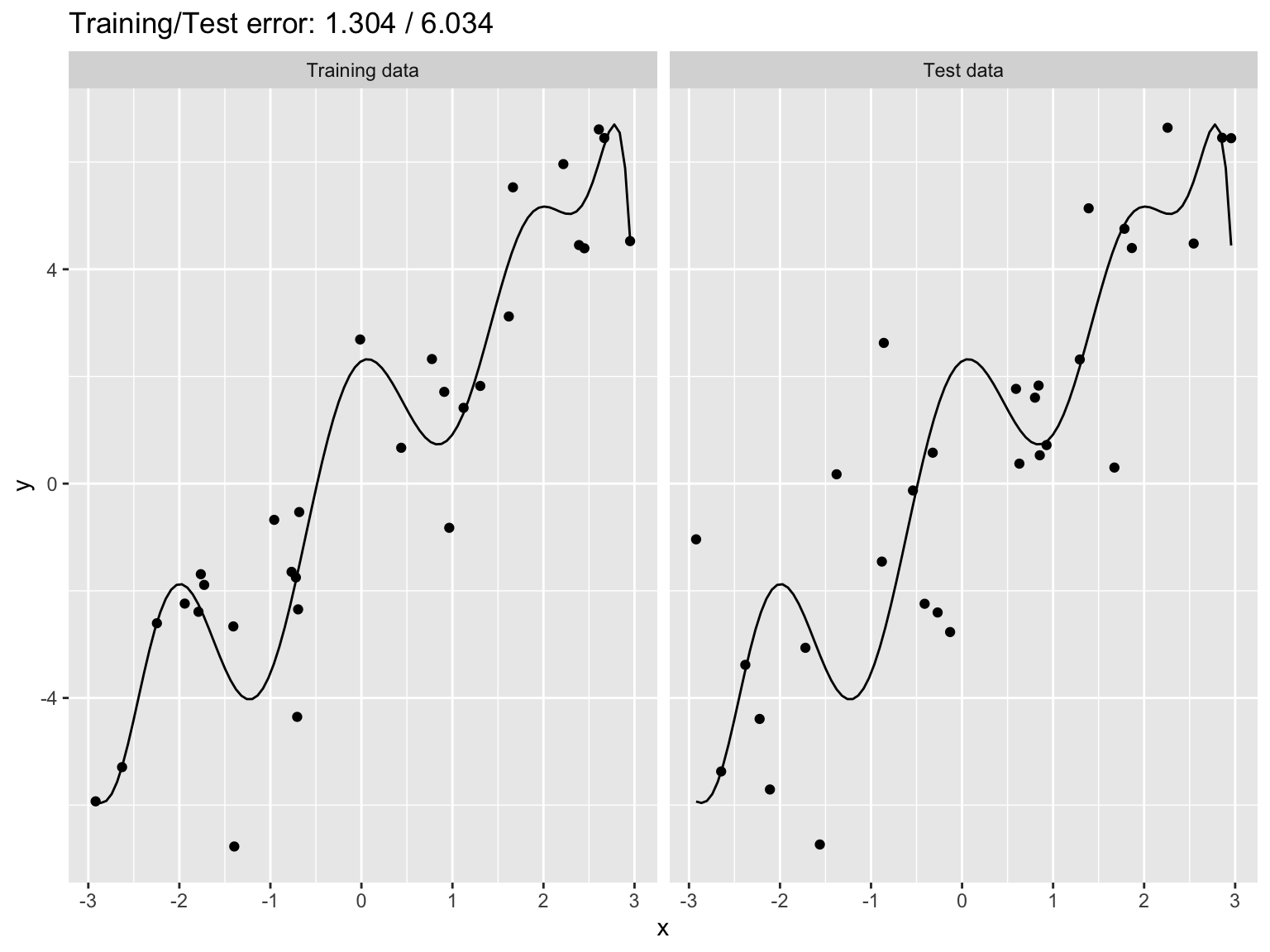# Part II

Sample-splitting and cross-validation

# Sample-splitting

Given a data set, how can we estimate test error? (Can’t simply simulate more data for testing.) We know training error won’t work

A tried-and-true technique with an old history in statistics: sample-splitting

• Split the data set into two parts
• First part: train the model/method
• Second part: make predictions
• Evaluate observed test error

# Examples

xy_data <- read.table("https://raw.githubusercontent.com/benjaminleroy/36-350-summer-data/master/Week6/xy.dat")
head(xy_data)
##           x         y
## 1 -2.908021 -7.298187
## 2 -2.713143 -3.105055
## 3 -2.439708 -2.855283
## 4 -2.379042 -4.902240
## 5 -2.331305 -6.936175
## 6 -2.252199 -2.703149
dim(xy_data)
##  50  2
n <- nrow(xy_data)

# Split data in half, randomly
set.seed(0)
xy_data$inds <- factor(sample(rep(1:2, length = n)), levels = 1:2, labels = c("Training data", "Test data")) head(xy_data$inds, 10)
##   Training data Test data     Test data     Training data Test data
##   Test data     Test data     Training data Test data     Test data
## Levels: Training data Test data
table(xy_data$inds) ## ## Training data Test data ## 25 25 ggplot(xy_data) + geom_point(aes(x = x, y = y, color = inds)) + labs(color = "Data")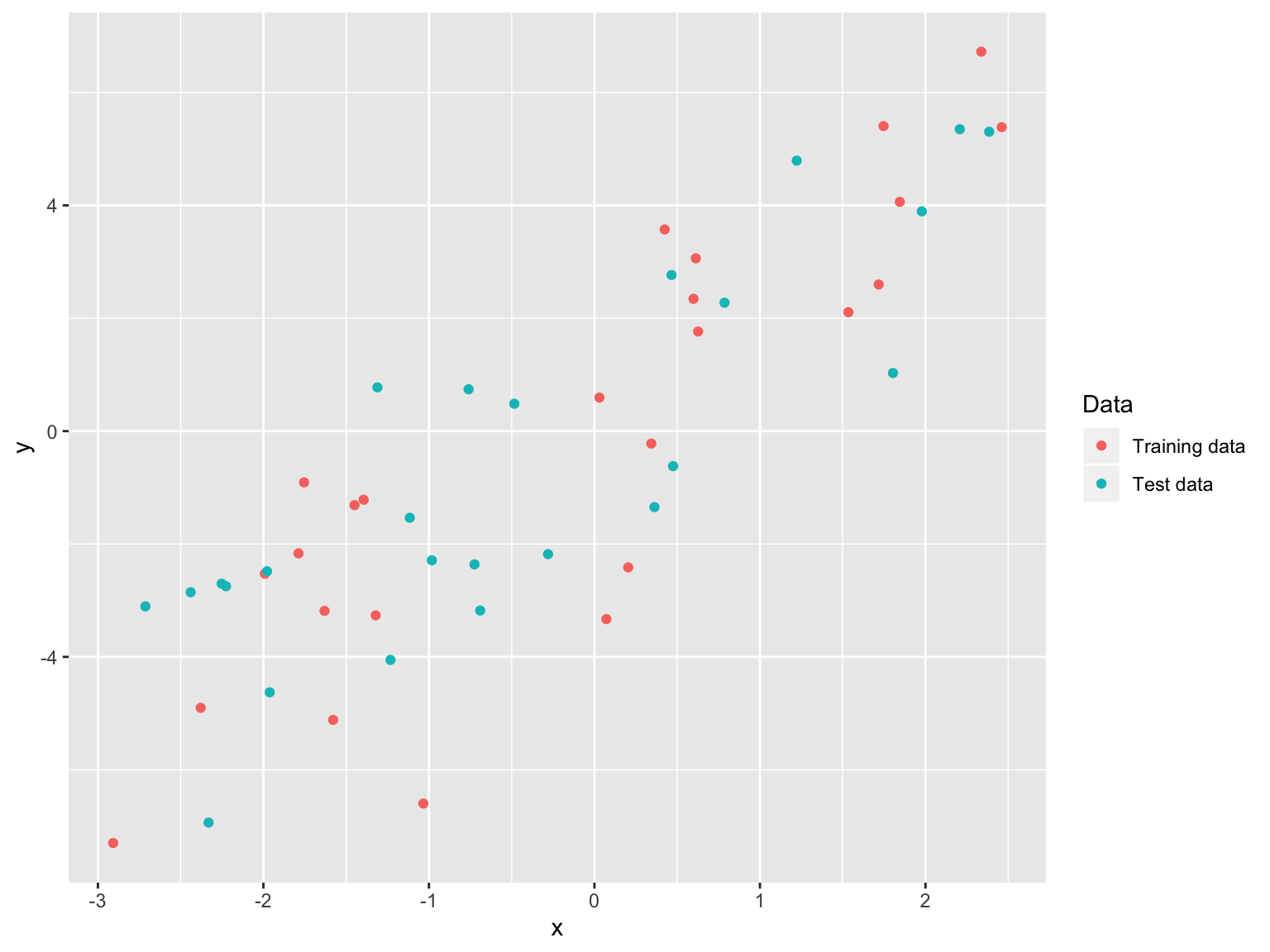# Train on the first half lm_1 <- xy_data %>% filter(inds == "Training data") %>% lm(y ~ x, data = .) lm_10 <- xy_data %>% filter(inds == "Training data") %>% lm(y ~ poly(x, 10), data = .) # Predict on the second half, evaluate test error pred_1 <- xy_data %>% filter(inds == "Test data") %>% predict(lm_1, .) pred_10 <- xy_data %>% filter(inds == "Test data") %>% predict(lm_10, .) test_err_1 <- mean((xy_data$y[xy_data$inds == "Test data"] - pred_1)^2) test_err_10 <- mean((xy_data$y[xy_data$inds == "Test data"] - pred_10)^2) # Plot the results xx <- seq(min(xy_data$x), max(xy_data$x), length = 100) data_vis_lm <- data.frame(x = rep(xx, 2), pred = c(predict(lm_1, data.frame(x = xx)), predict(lm_10, data.frame(x = xx))), tt = factor(rep(1:2, each = 100), levels = 1:2, labels = c("Linear", "Poly(10)"))) ggplot(data_vis_lm) + geom_point(data = xy_data, aes(x = x, y = y, color = inds)) + geom_line(data = data_vis_lm, aes(x = x, y = pred, color = tt)) + labs(title = paste0("Test error (Linear / Poly(10)): ", round(test_err_1,3) ," / ", round(test_err_10,3))) + facet_grid(~tt) + labs(color = "Line model type or Data Type") + theme_minimal() + theme(legend.position = "bottom") + scale_color_manual(values = c("red", "blue", "grey", "black"))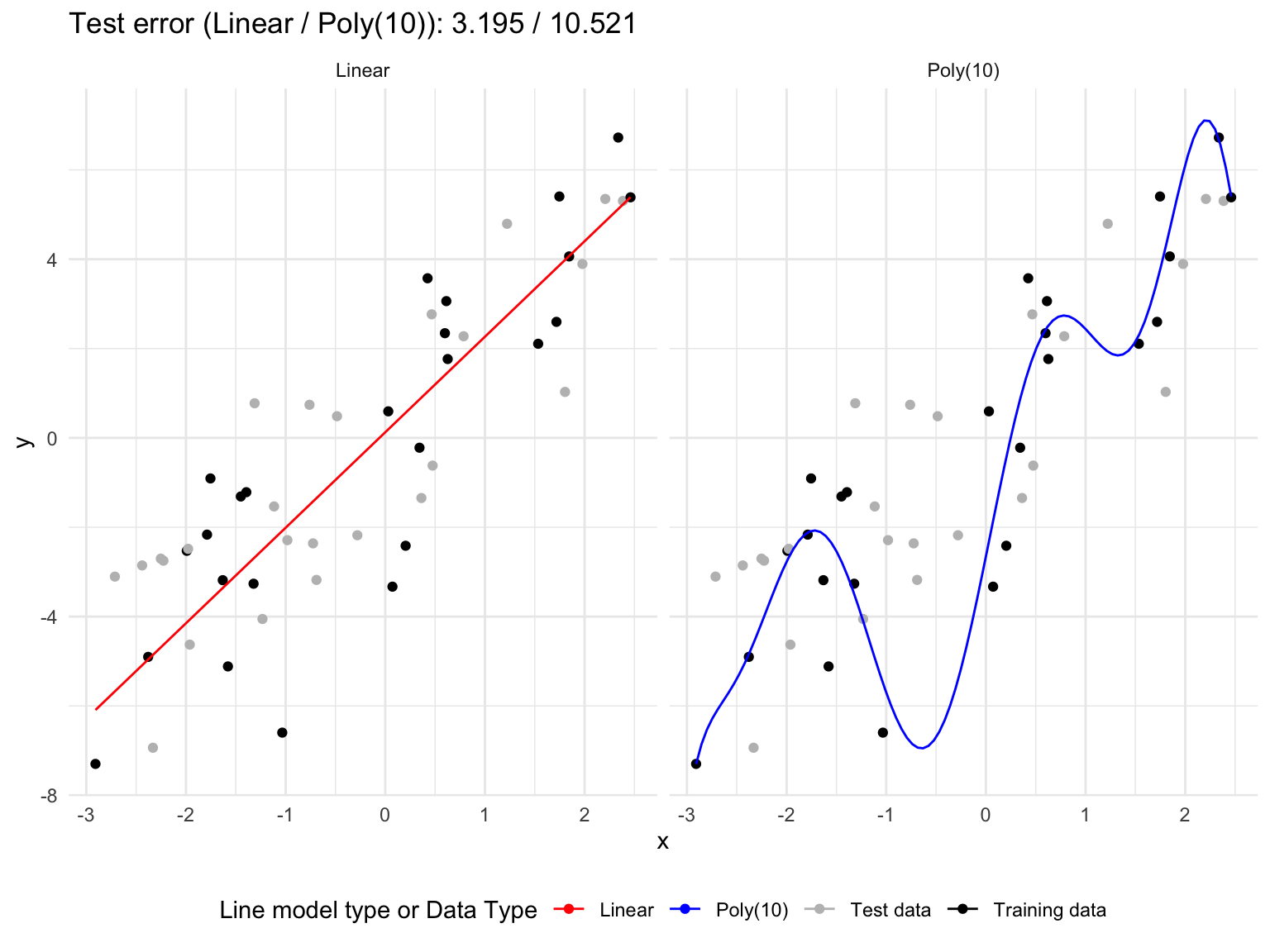# Cross-validation Sample-splitting is simple, effective. But its it estimates the test error when the model/method is trained on less data (say, roughly half as much) An improvement over sample splitting: $$k$$-fold cross-validation • Split data into $$k$$ parts or folds • Use all but one fold to train your model/method • Use the left out folds to make predictions • Rotate around the roles of folds, $$k$$ rounds total • Compute squared error of all predictions, in the end A common choice is $$k=5$$ or $$k=10$$ (sometimes $$k=n$$, called leave-one-out!)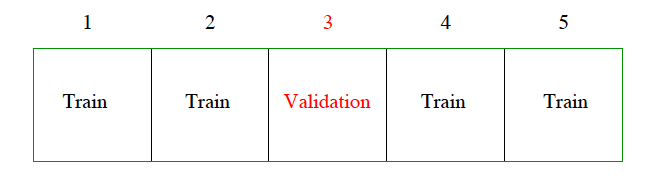For demonstration purposes, suppose $$n=6$$ and we choose $$k=3$$ parts Data point Part Trained on Prediction $$Y_1$$ 1 2,3 $$\hat{Y}^{-(1)}_1$$ $$Y_2$$ 1 2,3 $$\hat{Y}^{-(1)}_2$$ $$Y_3$$ 2 1,3 $$\hat{Y}^{-(2)}_3$$ $$Y_4$$ 2 1,3 $$\hat{Y}^{-(2)}_4$$ $$Y_5$$ 3 1,2 $$\hat{Y}^{-(3)}_5$$ $$Y_6$$ 3 1,2 $$\hat{Y}^{-(3)}_6$$ Notation: model trained on parts 2 and 3 in order to make predictions for part 1. So prediction $$\hat{Y}^{-(1)}_1$$ for $$Y_1$$ comes from model trained on all data except that in part 1. And so on The cross-validation estimate of test error (also called the cross-validation error) is $\frac{1}{6}\Big( (Y_1-\hat{Y}^{-(1)}_1)^2 + (Y_1-\hat{Y}^{-(1)}_2)^2 + (Y_1-\hat{Y}^{-(2)}_3)^2 + \\ (Y_1-\hat{Y}^{-(2)}_4)^2 + (Y_1-\hat{Y}^{-(3)}_5)^2 + (Y_1-\hat{Y}^{-(3)}_6)^2 \Big)$ # Examples # Split data in 5 parts, randomly k <- 5 set.seed(0) xy_data$inds_cv <- sample(rep(1:k, length=n))
head(xy_data$inds_cv, 10) ##  5 4 3 2 2 5 5 1 3 1 table(xy_data$inds_cv)
##
##  1  2  3  4  5
## 10 10 10 10 10
# Now run cross-validation: easiest with for loop, running over
# which part to leave out
pred_mat <- matrix(0, n, 2) # Empty matrix to store predictions
for (i in 1:k) {
cat(paste("Fold",i,"... "))

data_train <- xy_data[xy_data$inds_cv != i,] # Training data data_test <- xy_data[xy_data$inds_cv == i,] # Test data

# Train our models
lm_1_minus_i <- lm(y ~ x, data = data_train)
lm_10_minus_i <- lm(y ~ poly(x,10), data = data_train)

# Record predictions
pred_mat[xy_data$inds_cv == i,1] <- predict(lm_1_minus_i, data.frame(x = data_test$x))
pred_mat[xy_data$inds_cv == i,2] <- predict(lm_10_minus_i, data.frame(x = data_test$x))
}
## Fold 1 ... Fold 2 ... Fold 3 ... Fold 4 ... Fold 5 ...
# Compute cross-validation error, one for each model
cv_errs <- colMeans((pred_mat - xy_data$y)^2) # xx <- seq(min(xy_data$x), max(xy_data$x), length = 100) data_vis_lm <- data.frame(x = xx, pred_1 = predict(lm_1, data.frame(x = xx)), pred_10 = predict(lm_10, data.frame(x = xx))) # Plot the results vis_lm <- ggplot(xy_data) + geom_point(aes(x = x, y = y, color = factor(inds_cv))) + geom_line(data = data_vis_lm, aes(x = x, y = pred_1), color = "black") + labs(color = "Fold index:", #title = "Cross-validation vs Full Fit", subtitle = paste("(Linear) CV error:", round(cv_errs,3))) vis_poly <- ggplot(xy_data) + geom_point(aes(x = x, y = y, color = factor(inds_cv))) + geom_line(data = data_vis_lm, aes(x = x, y = pred_10), color = "black") + labs(color = "Fold index:", #title = "Cross-validation vs Full Fit", subtitle = paste("Poly(10) CV error:", round(cv_errs,3))) library(gridExtra) grid.arrange(vis_lm, vis_poly, ncol = 2, top = "Cross-validation vs Full Fit")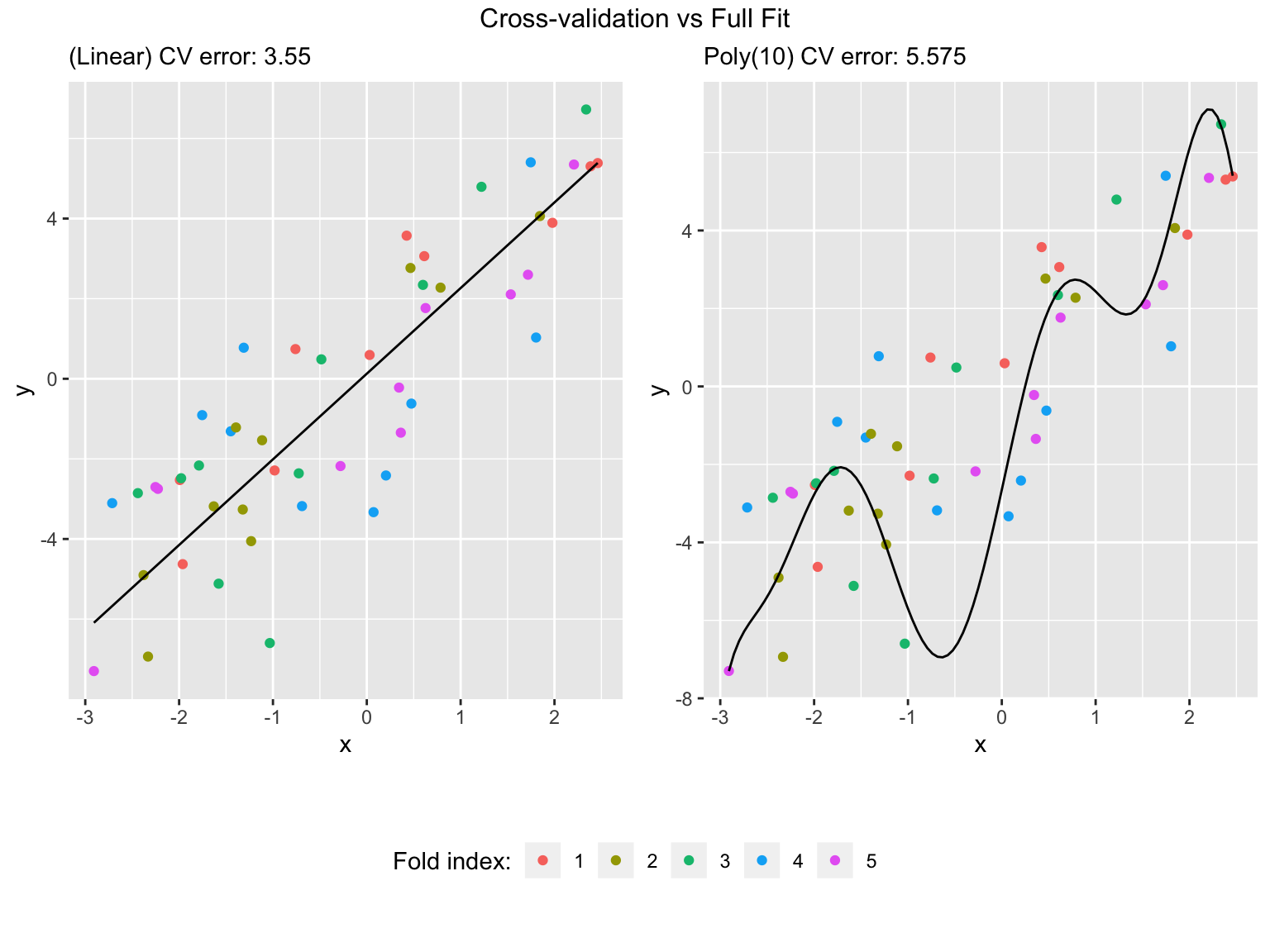# Now we visualize the different models trained, one for each CV fold data_vis_1 <- data.frame(x = xx) data_vis_10 <- data.frame(x = xx) for (i in 1:k) { cat(paste("Fold",i,"... ")) data_train <- xy_data[xy_data$inds_cv != i,] # Training data
data_test <- xy_data[xy_data\$inds_cv == i,] # Test data

# Train our models
lm_1_minus_i <- lm(y ~ x, data = data_train)
lm_10_minus_i <- lm(y ~ poly(x,10), data = data_train)

# Record predictions
data_vis_1 <- cbind(data_vis_1